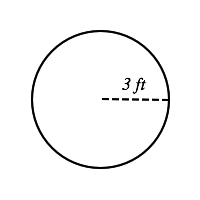### Test: SAT II Math I1.

# Find the diameter, circumference and area of the circle above.

Diameter= 3ft

Circumference=37.68 ft

Area= 28.7 ft2

Diameter= 6 ft

Circumference= 19 ft

Area= 30 ft2

Diameter= 9ft

Circumference=37.68 ft

Area= 28 ft2

Diameter= 6 ft

Circumference=18.84 ft

Area= 28.27 ft2

Diameter= 6ft

Circumference=37.68 ft

Area= 28.27 ft2

1/2 questions

0%

## Access results and powerful study features!

Take 15 seconds to create an account.